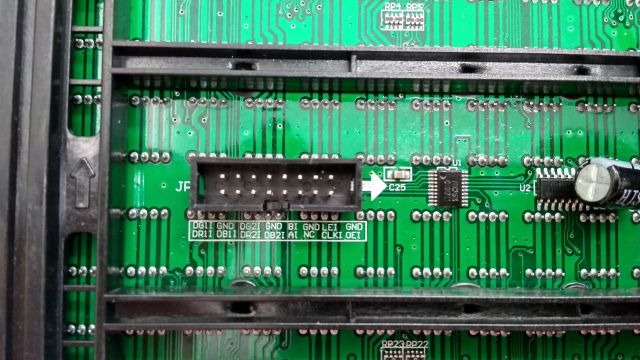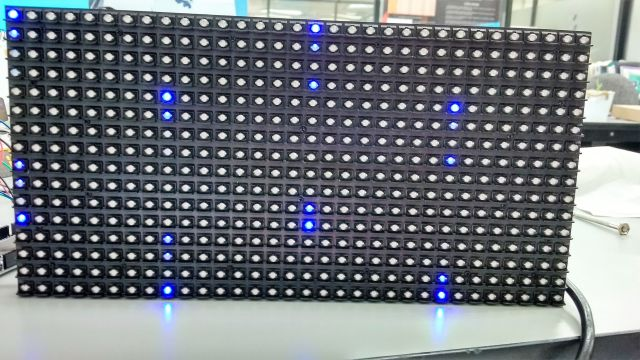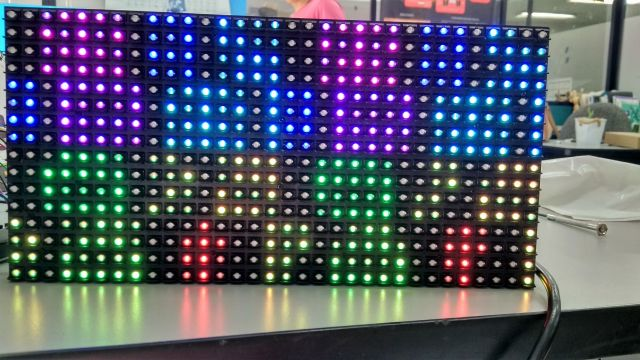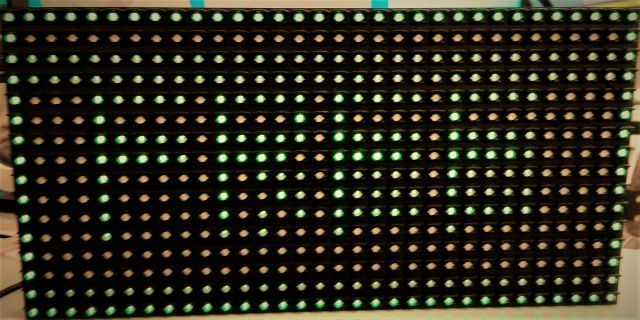Go Down

Topic: Problem with RGB panel 16x32  (Read 593 times)previous topic - next topic

chempiDec 13, 2018, 05:13 pm
Hi

I am trying to use a RGB panel, I am using an arduino MEGA 2560 and the adafruit libraries. The problem is that the matrix is not showing what I send to it, for instance the example that should show "16x32 *RGB*", showed a bunch of disordered pixel of several colors (photo), I also tried to turn on 4 single pixels (the corners), but several of them turn on, and not the pixels that sent. (photo). There is a picture of the led matrix that I am using.

I am following this pinout

DR1I -> 24
DG1I -> 25
DP1I -> 26
DR2I -> 27
DG2I -> 28
DP2I -> 29
AI -> A0
BI -> A1
CN -> A2
LEI -> 10
CLKI -> 11
OEI -> 9
All GND to GND

I appreciate your help

chempi#1
Dec 13, 2018, 05:44 pm
Attached pictures of my problem.chempi#2
Dec 13, 2018, 09:34 pm
After reading several post, I figure out that I have a 32x16 p10 matrix, that only has A and B pins, no C pin, there is any modification to the adafruit library or another library in order to use my panel?

Regards

chempi#3
Dec 14, 2018, 05:11 pmLast Edit: Dec 14, 2018, 05:11 pm by chempi
Hello, after trying a lot, I thing that I discover the pattern for the 32x16 p10, I used the following code:

Code: [Select]
//**************************************************************//
// Following codes simply shifts out data for 16x32 LED matrix.
// shifts data turning on one led of a time, to see the pattern of
// the led matrix
// For ARDUINO MEGA 2560
//****************************************************************

//Pins declaration
int LP = 10;            // Latch Pin
int ClkP = 11;          // Clock Pin
int R1P = 24;           // R1 Pin
int G1P = 25;           // B1 Pin
int B1P = 26;           // G1 Pin
int R2P = 27;           // R2 Pin
int G2P = 28;           // B2 Pin
int B2P = 29;           // G2 Pin
int AP = A0;            // A Pin
int BP = A1;            // B Pin
int CP = A2;            // C Pin
int OEP = 9;            // OE Pin
byte col=0;

void setup() {
//Set pins to output so you can control the shift register
pinMode(LP, OUTPUT);
pinMode(ClkP, OUTPUT);
pinMode(R1P, OUTPUT);
pinMode(B1P, OUTPUT);
pinMode(G1P, OUTPUT);
pinMode(R2P, OUTPUT);
pinMode(B2P, OUTPUT);
pinMode(G2P, OUTPUT);
pinMode(AP,OUTPUT);
pinMode(BP,OUTPUT);
pinMode(CP,OUTPUT);
pinMode(OEP,OUTPUT);
pinMode(13,OUTPUT);
digitalWrite(AP, LOW);
digitalWrite(BP, LOW);
digitalWrite(CP, LOW);
digitalWrite(OEP, LOW);
digitalWrite(ClkP, LOW);
randomSeed(112);
//Initialize the column counter, sending the last data first
col=63;
}

void loop() {
//Function that only sends the Red bit to the half top panel
shiftOut1(1,0,0,0,0,0);
digitalWrite(OEP, HIGH);
//Only send data to the fist address A0 B0
digitalWrite(AP, 0);
digitalWrite(BP, 0);
//Enable outputs
digitalWrite(OEP, LOW);
//Delay in order to see the pattern
delay(100);
//Counter for columns
col--;
//Resets the counter
if ( col == 255) col =63;
}

//Function to send 64 data to the led matrix
void shiftOut1(byte cr1, byte cg1, byte cb1, byte cr2, byte cg2, byte cb2)
{
uint8_t i;

digitalWrite(LP, HIGH);
//Only sends data when the counter its equal to the col variable from the loop
for (i = 0; i < 64; i++)  {
if (i==col) {
digitalWrite(R1P, cr1);
digitalWrite(G1P, cg1);
digitalWrite(B1P, cb1);
digitalWrite(R2P, cr2);
digitalWrite(G2P, cg2);
digitalWrite(B2P, cb2);
}
// For all the data it sends 0 to all colors
else {
digitalWrite(R1P, 0);
digitalWrite(G1P, 0);
digitalWrite(B1P, 0);
digitalWrite(R2P, 0);
digitalWrite(G2P, 0);
digitalWrite(B2P, 0);
}
//Clock cycle
digitalWrite(ClkP, HIGH);
digitalWrite(ClkP, LOW);
//Latch data
digitalWrite(LP, LOW);
}
}

Attached is the pattern that I found

I also modified the excel sheet that is posted in another post in order to work with this panel, but when I tried to send it to my panel, I noticed some mistakes in the image.

The code that I am using to send the data is:

Code: [Select]

//**************************************************************//
// Following codes simply shifts out data for 16x32 LED matrix.
//****************************************************************
uint64_t row1;
uint64_t temp;
int LP = 10;            // Latch Pin
int ClkP = 11;           // Clock Pin
int R1P = 24;          // R1 Pin
int G1P = 25;          // B1 Pin
int B1P = 26;           // G1 Pin
int R2P = 27;           // R2 Pin
int G2P = 28;           // B2 Pin
int B2P = 29;           // G2 Pin
int AP = A0;            // A Pin
int BP = A1;            // B Pin
int CP = A2;            // C Pin
int OEP = 9;          // OE Pin
int row = 0;

void setup() {
//set pins to output so you can control the shift register
pinMode(LP, OUTPUT);
pinMode(ClkP, OUTPUT);
pinMode(R1P, OUTPUT);
pinMode(B1P, OUTPUT);
pinMode(G1P, OUTPUT);
pinMode(R2P, OUTPUT);
pinMode(B2P, OUTPUT);
pinMode(G2P, OUTPUT);
pinMode(AP,OUTPUT);
pinMode(BP,OUTPUT);
pinMode(CP,OUTPUT);
pinMode(OEP,OUTPUT);

digitalWrite(AP, LOW);
digitalWrite(BP, LOW);
digitalWrite(CP, LOW);
digitalWrite(LP, LOW);
digitalWrite(OEP, LOW);
row=0;
temp = 0x0000000000000001;

row1=0xFF8FFFBCFFFBFFE1;
row1=0x0188002200828001;
row1=0x0188002200828001;
row1=0x018F003C00F380C1;
row1=0x1180140041008001;
row1=0x1180240041008001;
row1=0x11804400DF008701;
row1=0x01FF00FF00FF80FF;

}

byte i;
void loop() {
for(row=0; row<4; row++){
for (i = 0; i < 64; i++)  {
digitalWrite(B1P, 0);
digitalWrite(G1P, !!(row1[row] & (temp << (63-i))));
digitalWrite(R1P, 0);
digitalWrite(B2P, 0);
digitalWrite(G2P, !!(row1[row+4] & (temp << (63-i))));
digitalWrite(R2P, 0);
digitalWrite(ClkP, HIGH);
digitalWrite(ClkP, LOW);
}
digitalWrite(OEP, HIGH);
digitalWrite(LP, HIGH);
digitalWrite(AP, row & B1);
digitalWrite(BP, row & B10);
digitalWrite(OEP, LOW);
digitalWrite(LP, LOW);
}
}

Image with problemsI appreciate your help

Regards

chempi#4
Dec 14, 2018, 06:47 pm
Video of the pattern

chempi#5
Dec 14, 2018, 08:45 pm
Test with some shapes

There is some mixing on the edges of the square, but I dont know why is happenning.

chempi#6
Jan 17, 2019, 01:10 pmLast Edit: Jan 17, 2019, 01:10 pm by chempi
Finally I managed to make it work, but no with the RGB Library, but using the ports to send the data to the panel. The library from adafruit can output the generated matrix from the library, I created a sheet to transform the output to get the info to plot it in the panel, It is here is anybody is interested on it.

In order to get the matrix dump use the following command.

matrix.dumpMatrix();

Here is an example

// testshapes demo for Adafruit RGBmatrixPanel library.
// Demonstrates the drawing abilities of the RGBmatrixPanel library.
// For 16x32 RGB LED matrix:

// Written by Limor Fried/Ladyada & Phil Burgess/PaintYourDragon
// for Adafruit Industries.
// BSD license, all text above must be included in any redistribution.

#include <RGBmatrixPanel.h>

// Most of the signal pins are configurable, but the CLK pin has some
// special constraints.  On 8-bit AVR boards it must be on PORTB...
// Pin 8 works on the Arduino Uno & compatibles (e.g. Adafruit Metro),
// Pin 11 works on the Arduino Mega.  On 32-bit SAMD boards it must be
// on the same PORT as the RGB data pins (D2-D7)...
// Pin 8 works on the Adafruit Metro M0 or Arduino Zero,
// Pin A4 works on the Adafruit Metro M4 (if using the Adafruit RGB
// Matrix Shield, cut trace between CLK pads and run a wire to A4).

//#define CLK  8   // USE THIS ON ARDUINO UNO, ADAFRUIT METRO M0, etc.
//#define CLK A4 // USE THIS ON METRO M4 (not M0)
#define CLK 11 // USE THIS ON ARDUINO MEGA
#define OE   9
#define LAT 10
#define A   A0
#define B   A1
#define C   A2

RGBmatrixPanel matrix(A, B, C, CLK, LAT, OE, false);

void setup() {

matrix.begin();
Serial.begin(9600);
// print each letter with a rainbow color

matrix.setTextColor(matrix.Color333(7,7,0));
matrix.print('1');
matrix.setTextColor(matrix.Color333(0,7,0));
matrix.print('6');
matrix.setTextColor(matrix.Color333(0,0,7));
matrix.print('x');
matrix.setTextColor(matrix.Color333(7,0,7));
matrix.print('3');
matrix.setTextColor(matrix.Color333(0,7,7));
matrix.print('2');

matrix.setCursor(1, 9);  // next line
matrix.setTextColor(matrix.Color333(0,0,7));
matrix.print('*');
matrix.setTextColor(matrix.Color333(7,7,7));
matrix.print('R');
matrix.setTextColor(matrix.Color333(0,7,0));
matrix.print('G');
matrix.setTextColor(matrix.Color333(0,0,7));
matrix.print('B');
matrix.setTextColor(matrix.Color333(7,0,0));
matrix.print('*');
matrix.dumpMatrix();

// whew!
}

void loop() {
// Do nothing -- image doesn't change
}

chempi#7
Jan 17, 2019, 02:05 pm
Correction on the file.

Santa_Harleme#8
May 14, 2019, 08:33 am
Hello,
Can you give me the final code please.

Go Up